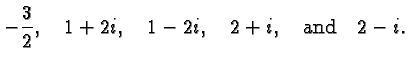# Mathematics 1010 online

## Factoring and Solving Polynomial Equations

It's obvious that a polynomial such as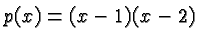is zero whenor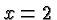. The same fact is less obvious when we rewrite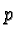as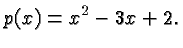In the first form,is said to be factored It is easier to tell the roots of a polynomial if it is factored, but it is also possible to go the other direction: to factor a polynomial when we know one or more of its roots. Just how to go about this is described on this page.

### Knowing a Real Root

Suppose we recognize, for example by trial and error, or drawing a graph, that the polynomial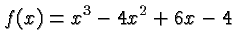is zero when. This can be easily checked: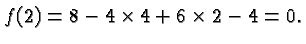The fact that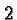is a root implies that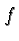can be written as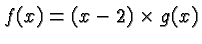where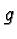is a polynomial of degree. As discussed elsewhere,can be found by synthetic division :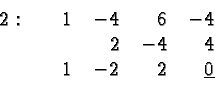This means that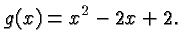To find the remaining zeros ofwe only have to find the zeros of. This means we have to solve the quadratic equation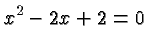which has the solutions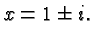Thus we see that the roots ofareand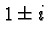, and we were able to find all of them once we recognized one of them. In general, if we have a polynomialand one real root we can use synthetic division to obtain a polynomial of degree one less whose roots equal the remaining roots of.

### Knowing a Complex Root

Suppose the complex number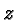is a root of a polynomial. Then we can write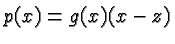just as above. However, the coefficients ofare complex numbers even if the coefficients ofare real numbers. The process still works, but it requires complex arithmetic, and there is a better way that uses real arithmetic only.

The key fact in this context is that conjugate complex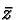ofis also a root of. It's a simple exercise to see that this is true by observing that the conjugate complex of a real number is the number itself, and that for any complex numbers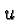and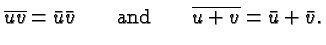Thus if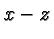is a factor ofthen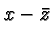is also a factor, and so is the product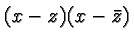. This last term is a quadratic polynomial whose coefficients are real. To see this let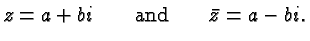Then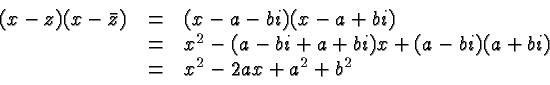Hence if we know that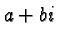is a root ofthen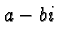is also a root and we can obtain a polynomial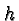satisfying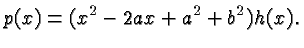The degree ofisless than the degree of.

Let's look again at the above example. Essentially we work it backwards. Again, letWe know that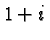is a root of. Thus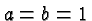and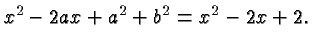So we can write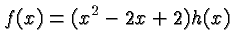whereis a linear polynomial. It can be obtained by long division, and we know from the above discussion that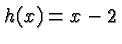### A Comprehensive Example

The following example shows all the principles described above in action. It does not address the question of how one might find a particular root. The answer to that question depends on the context in which the polynomial in question occurs. There are general purpose methods for finding a single root but they are beyond the scope of this class.

Let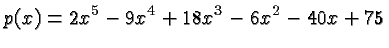and suppose that we wish to find all roots of.

It is easily checked that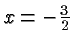is a root of. This means that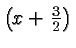is a factor of. We find it by synthetic division :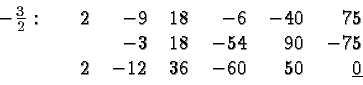Hence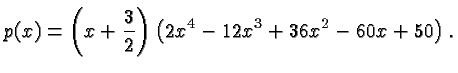Note that the remainder of the division byis zero (underlined in the above table) which confirms thatis in fact a root.can be rewritten (after multiplying first factor and dividing the second factor by 2) as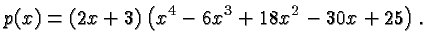We now need to find the roots of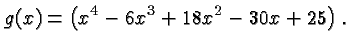It turns out that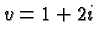is a root of.

Thus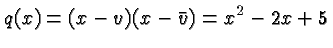is a factor of. We find the quotient by long division :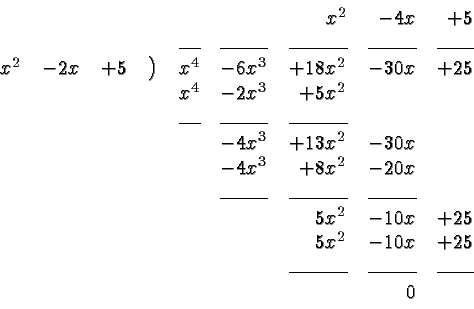Again, the fact that the remainder is zero indicates that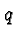is actually a factor of. In fact,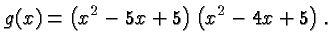The roots of the first factor are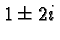, that's how we constructed that factor. The roots of the second factor can be found by solving the quadratic equation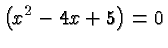which has the solutions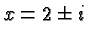. The roots of our original polynomialare, therefore: